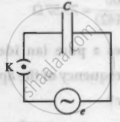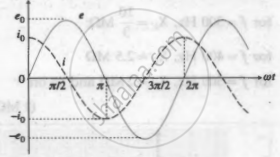# An AC source generating a voltage e = e0sinωt is connected to a capacitor of capacitance C. Find the expression for the current i flowing through it. Plot a graph of e and i versus ωt. - Physics

An AC source generating a voltage e = e0sinωt is connected to a capacitor of capacitance C. Find the expression for the current i flowing through it. Plot a graph of e and i versus ωt.

#### Solution

The following figure shows an AC source, generating a voltage e = e0 sin wt, connected to a capacitor of capacitance C. The plates of the capacitor get charged due to the applied voltage. As the alternating voltage is reversed in each half cycle, theAn AC source connected to a capacitor

capacitor is alternately charged and discharged. If q is the charge on the capacitor, the corresponding potential difference across the plates of the capacitor is V = "q"/"C" ∴ q = CV. q and V are functions of time, with V = e = e0 sin ωt.

The instantaneous current in the circuit is i = "dq"/"dt" = "d"/"dt" ("CV") = "C" "dV"/"dt"

= "C" "d"/"dt" ("e"_0 sin omega"t") = omega "C""e"_0 cos omega"t"

∴ i = "e"_0/((1//omega"C")) sin (omega"t" + pi/2) = "i"_0 sin (omega"t" + pi/2)

where "i"_0 = "e"_0/(1//omega"C") is the peak value of the current.

 ωt (rad) ωt + π/2 (rad) e = e0 sin ωt i = i0 sin (omega"t" + pi/2) 0 pi/2 0 i0 pi/2 π e0 0 π (3pi)/2 0 - i0 (3pi)/2 2π - e0 0 2π 2π + pi/2 0 i0

The above table shows gives the values of e and i for different values of ωt and the following figure shows graphs of e and i versus ωt. i leads e by a phase angle of π/2 rad.Graphs of t and i venus wt for a purely capacitive AC circuit

Concept: Power in Ac Circuit
Is there an error in this question or solution?

#### APPEARS IN

Balbharati Physics 12th Standard HSC Maharashtra State Board
Chapter 13 AC Circuits
Exercises | Q 11 | Page 305